Courses

# Chapter 1 Introduction - RAC (Refrigeration and Air Conditioning), Mechanical Engineering Mechanical Engineering Notes | EduRev

## Mechanical Engineering : Chapter 1 Introduction - RAC (Refrigeration and Air Conditioning), Mechanical Engineering Mechanical Engineering Notes | EduRev

The document Chapter 1 Introduction - RAC (Refrigeration and Air Conditioning), Mechanical Engineering Mechanical Engineering Notes | EduRev is a part of the Mechanical Engineering Course Mechanical Engineering SSC JE (Technical).
All you need of Mechanical Engineering at this link: Mechanical Engineering

INTRODUCTION

• Unit of Refrigerating Capacity is TR (Tonne Refrigeration)

1 TR = Rate of removal of heat from 1 tonne of water to freeze it into ice in 24 hr at 0ºC = 50.4 K Cal/min

•  1 kCal = 4.18 KJ

Specific heat of water =4.18 KJ/kgK

Specific heat of ice = 2.11 KJ/kgK

Specific heat of vapur = 1.99 KJ/kgK

Latent heat water

in fusion = 335 KJ/kg (at 0ºC)

in vapourization = 2260 KJ/kg (at 100ºC)PRODUCTION OF LOW TEMPERATURE

(i) Throttling expansion of liquid with flashing

(ii) Reversible adiabatic expansion of a gas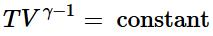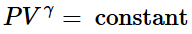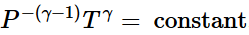(iii) Irreversible adiabatic expansion of real gasIn contrast to the reversible expansion, an irreversible expansion is not performed continuously at equilibrium by means of infinitesimal changes in pressure. In the limiting case, the value of the pressure p abruptly changes from p1 and p2. The following expansion proceeds in one step against a constant pressure p2. Hence,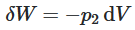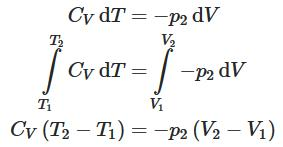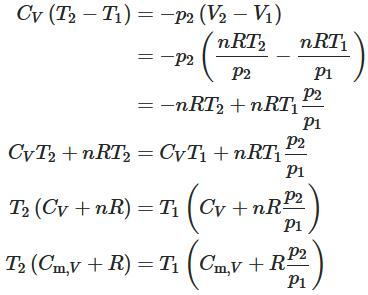(iv) Thermoelectric effect or peltier effect

SATURATION PRESSURE VS. SATURATION TEMPERATURE LINE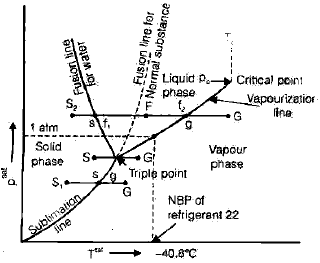•  A refrigerating machines is a device which transfers heat from a low temperature body to a high temperature body by consuming work.
•  Schematic Diagram of Refrigerating/ AC machine with temperature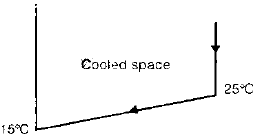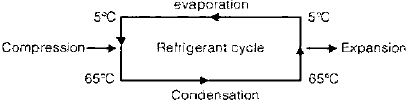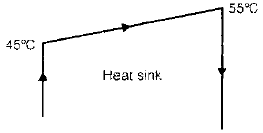•  The cooled space is to be maintained at 15ºC. The refrigerating machine takes the air at 25ºC, and due to evaporation of refrigerant, heat is taken from the air which is cooled to 15ºC and returned back to cooled space. During compression, the temperature rises to 65º while outside temperature is 45º. Hence heat is transferred to outside air while the refrigerant is condensed and temperature of outside air is raised. This condensed /liquid refrigerant is expended by a throttling device and temperature lowers again to 5ºC.

Units in Refrigeration

1 cal = 4.1868 Joule (J)

1 horse power = 746 Watt (W)

1 unit of power = 1 KWH

= 3600 kJ = 860 K cal

1 TR (ton refrigeration) = 50 K cal/min

= 3.5167 kW = 211 KJ/min.

Properties of Air

CP = 1.005 KJ/kgK

Cv = 0.718 KJ/kgK

R = 0.287 KJ/ kgK

g = 1.4

M = 28.966

REFRIGERATIN MACHINE/HEAT PUMP

•  It contains evaporator, compressor, condenser, expander.
•  The processes involved in the cycle are as follows: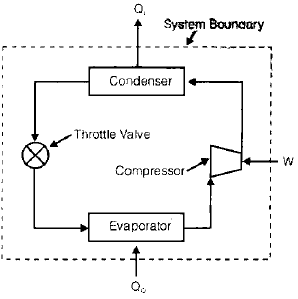•  Heat Q0 is absorbed in the evaporator by the evaporation of a liquid refrigerant at a low pressure P0 and corresponding low saturation temperature T0
•  The evaporated refrigerant vapour is Compressed to a high pressure Pk in the compressor consuming work W.
•  Heat Qk is rejected from the condenser to the surrounding.
• When refrigerating machine is used for cooling the space, it is called refrigerator. When the machine is used for heating the space, it is called heat pump.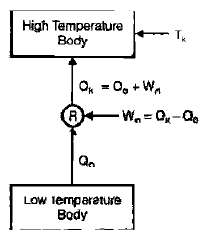COEFFICIENT OF PERFORMANCE (COP)

COP = Energy Ratio  =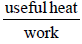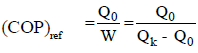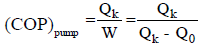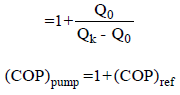(COP)pump =1+(COP)ref

•   If refrigerator /heat pump cycle is reversed, it becomes a heat engine.
•   Thermal efficiency of heat engine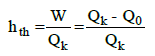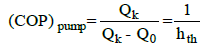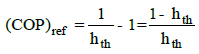COP of Different Vapour Compression SystemType of vapour compression systemCOPWater cooled3Air Cooled2Domestic Refrigerator1Vapour absorption system< 1• Power consumption of a refrigerator /heat pump

1 H.P.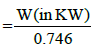Refrigerating capacity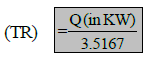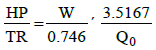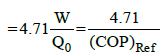where, W is power consumption (KW)

Q0 is refrigerating effect (KW)HEAT PUMP VS. ELECTRIC RESISTANCE HEATER

•  If 'W' is the energy consumption in electric resistance heater, its output is equal to W.
•   If 'W' is the energy consumption of heat pump. Its output

Qk = (COP)pump W = (1 + COP ref) W

•  COPref varies from 0 to ¥
•  COPpump varies from 1 to ¥
•  When refrigeration/Heat pump cycle is reversed carnot cycle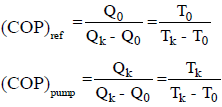•  For high COP for refrigerator or heat pump
•   T0 should be as high as possible
•  Tk Should be as low as possible
•  Water is a better cooling medium that air (i.e. higher COP & lower power consumption for water in refrigerator ) because, T0 = Evaporator Tk = Heat rejection temperature
•  Specific heat of water is about four times that air. [For same heat output (Qk) rise in water temperature is lower than air temperature (i.e low Tk)].
• Water has high thermal conductivity than air So for same temperature difference (refrigerant & air) and (refrigerant & water), more heat can be transferred in water system.
• so for small refrigeration system (A.C, Domestic refrigerator), air as cooling medium is used
• For big refrigeration system (Central A.C., ice plant, cold storage) water as cooling medium used.

VAPOUR AS REFRIGERANT IN A REVERSED CARNOT CYCLE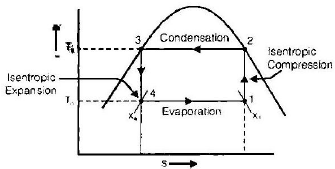For per Unit Mass of Vapour

Refrigeratinrg Effect = q0 = h1 – h4

Heat reject qm = h2 – h3

Compressor work Wc (Consumed )= h2 – h1

Expander work We (gained ) = h3 – h 4

Net Work = Wc – We = (h2 – h1) – (h3 – h4)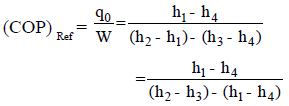GAS AS REFRIGERANT IN REVERSED CARNOT CYCLE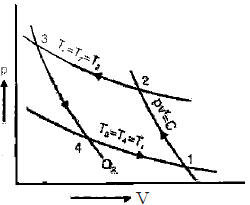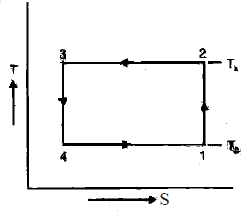Process 1-2: Reversible Adiabatic CompressionThis process is isentropic. The engine is perfect insulated so that no heat is lost and absorbed. Gas is compressed slowly until the temperature rises from TL to TH.Process 2-3: Reversible Isothermal Compression (TH=constant)During this process, heat is rejected. Gas is compressed reversibly at the constant temperature TH.Process 3-4: Reversible Adiabatic ExpansionThis process is isentropic. The engine is perfect insulated so that no heat is lost and absorbed. Gas expands slowly until the temperature drops from TH to TL.Process 4-1: Reversible Isothermal Expansion (TL=constant)During this process, heat is absorbed. Gas is compressed reversibly at the constant temperature TH.The coefficient of performance (COP) of any refrigerator or heat pump, reversible or irreversible, can be calculated with the general expression:

• For a Carnot refrigerator: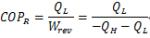Therefore

• For a Carnot refrigerator: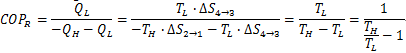The net work done can be calculated by the area enclosed in 1-2-3-4.Draw back of using Air as Refrigerant in Reversed Carnot Cycle

•  Isothermal process of heat absorption and rejection being very slow is not possible.
•  P-v diagram for gas is very narrow so very high stroke volume is needed to  have desired refrigerating effect.

Draw Back of Using Vapour as Refrigerant in Reversed Carnot Cycle

1. Liquid refrigerant may be trapped in heat of cylinder and damage the compressor valves.

2. Liquid droplets may wash away the lubricating oil from the walls of compressor cylinder.

3. Expander is costly and the work gained in expander is not significant.

•  The carnot cycle is the most efficient between the given temperature limits (Tk & T0)
•   Second law efficiency or exergetic efficiency for cooling or heating is given as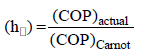Offer running on EduRev: Apply code STAYHOME200 to get INR 200 off on our premium plan EduRev Infinity!

83 docs|53 tests

,

,

,

,

,

,

,

,

,

,

,

,

,

,

,

,

,

,

,

,

,

,

,

,

;